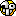# ForumCS2DScriptsTable within a table

#Table within a table

6 replies
Goto Page1Quattro
GAME BANNED
OfflineCan someone explain this test? I always use the array function to make a table and it works fine. But for some reason when I make tables within a table, all the tables are treated as one:
Code:
1
2
3
4
5
6
7
8
9
10
11
12
13
14
15
16
17
18
19
20
function array(size, value)
local array = {}
for i = 1, size do
array[i] = value
end
return array
end

test = array(5, array(5, 0))

test = test + 10
test = test + 10

print('Changed table:')
print(test)
print(test)

print('Unchanged table:')
print(test)
print(test)

The expected result:

Changed table:
10
10
Unchanged table:
0
0

Actual result:
Changed table:
10
10
Unchanged table:
10
10
TrialAndError
User
OfflineBecause the parameter "value" in the function array receives the same table and assigns the same table to every element.

You may think that the "array(5,0)" gets called seperately for every element but that's not the case.

Code:
1
2
3
4
5
6
7
8
function array(size, value)
local out = {}
for i = 1, size do
--value is the same for every element
out[i] = value
end
return out
end

the function array gets called twice:

-once for creating an array with 5 elements
-once for assigning the "array(5,0)" to every element within the table.

The code below is the behaviour you're looking for. Where every element inside "array2d" function gets assigned seperate memory address instead of all sharing one.

Code:
1
2
3
4
5
6
7
8
9
10
11
12
13
14
15
function array(size, value)
local out = {}
for i = 1, size do
out[i] = value
end
return out
end

function array2d(outer_size, inner_size, value)
local out = {}
for i = 1, outer_size do
out[i] = array(inner_size, value)
end
return out
end
edited 1×, last 05.08.19 04:34:04 pm
Quattro
GAME BANNED
Offline@TrialAndError:
It's easy without creating functions:
Code:
1
2
3
4
5
6
for i = 1, 100 do
array2D[i] = {}
for i2 = 1, 100 do
array2D[i][i2] = 0
end
end

I just don't understand why does it share the memory instead of creating separate tables? I thought they should be different functions with different local variablesTrialAndError
User
OfflineYeah, you could also do it like that, but the code would get longer the more table you add.

Okay, I'll try to explain how it shares.

So a table passed onto a function is always a reference (shallow copy).

Code:
1
2
3
4
5
6
7
8
9
--a function that expects a table
function func(tbl) end

--when passing a table into the function, the table isn't passed,
--but the memory address of where the table is:

--this will create a table somewhere in memory and pass the address to the function
func({1,2,3})
--what the function gets is something like (0x1ac1230)

Let's say we pass in the table: {0, 0, 0, 0, 0} (same as array(5,0)) as the value for the array function.

Code:
1
2
3
4
5
6
7
8
9
10
11
12
13
14
15
16
17
18
19
20
21
22
23
24
25
26
27
28
29
30
31
32
33
34
35
36
37
38
39
40
41
function array(size, value)
--the "value" parameter gets the value passed
--in this case {0, 0, 0, 0, 0}
--thus it will be the same as defining and initializing it here:
--local value = {0, 0, 0, 0, 0}

local out = {}

for i = 1, size do
--goes through each element and assign the value for them.
--if the value happens to be a table, then the memory address of "value"
-- is passed onto it which will be the same for every element because it's
out[i] = value
end

return out
end

--step for step:

array(5, array(5, 0))
-- 1. call the array function and pass on the arguments (first call to "array")
-- 2. when it gets to the "value" parameter, it will call array(5,0)
-- 3. array(5,0) gets called -> returns {0, 0, 0, 0, 0} (second call to "array")
-- 4. the value parameter gets assigned {0, 0, 0, 0, 0} somewhere in memory,
--let's say at memory (0x1ac1230).
-- 5. create a table to return (let's call it "out")
-- 6. loop through "size" amount of times
-- 7. for each element; point the element to the memory address of "value".
-- 8 return the table

--Total calls to "array" - 2
--Total created tables - 2

--array(5, array(5,0)) would be the same as:
local inner_array = array(5, 0)
array(5, inner_array)

--as you can see inner_array is passed onto array function as a reference or shallow copied
--thus all the elements would share the same memory address as inner_array
Gaios
Security Supporter
OfflineThe
value
parameter in
function array(size, value)
will be the same for all the sub-tables, because of you send the same table memory address to the function and execute the function's code.
Goo
User
OfflineUntested but wouldn't this work?

Code:
1
2
3
4
5
6
7
8
9
10
11
12
13
14
15
16
17
18
19
20
21
22
23
24
function get_new_table(inner_value)
return { inner_value }
end

function get_new_array(size, init_value, inner_value)
if size <= 0 then return end

local t, tbl

if type(init_value) == 'table' and next(init_value) == nil and inner_value then
t = true
end

for i = 1, size do
if t then
tbl[i] = get_new_table(inner_value)
else
tbl[i] = init_value
end
end
end

myArray = get_new_array(10, { }, 0)
Quattro
GAME BANNED
OfflineThank you, @TrialAndError: Finally I realized what's happeningIt's like copying tables, instead of making a deepcopy I made references to the same table.1﻿